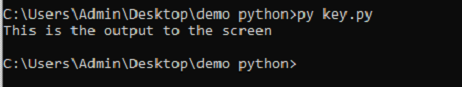# INPUT-OUTPUT IN PYTHON

#### INPUT-OUTPUT IN PYTHON

In Python, input() and print() functions are widely used for input and output operation.

The print() function is used to output data to the standard output device (screen). An example of its use is given below.

In the second print() statement, we can quickly notice that blank space was added between the string and the value of the variable, which is already made by default.

#### Example Code

print('This sentence is output on the screen')

#### OUTPUTAnother example is also given below:

#### CODE

a = 5 print('The value of a is', a)

#### OUTPUTIn the second print() statement, we can easily notice that blank space was added in between the string and the value of the variable which is already made by default.

#### Syntax of the print() function:

print(*objects, space =' ', end tab ='\n', file=sys.stdout, flush=False)
We use the “sep” separator to provide space between the values.
E.g., sep = * or sep = _. Here, objects are the value(s) to be printed. Space is entirely used as a separator between all the values.
After all the values have been printed, the endtab is printed, which takes into a new line. The file is
the only object where the codes are to be executed, and values are to be printed. Its default value is sys.stdout (screen).
We can format our output for attractive looks. This can be done with the help of the str.format() method.

By inputting values, we can make our program dynamic; for better flexibility
in our program, users can enter their input with the input() function.
E.g., n = input()
Or n = input(“Enter the String/number”)

We can take input with the help of some library functions like import math
print(math.pi) // Output:- 3.1415….